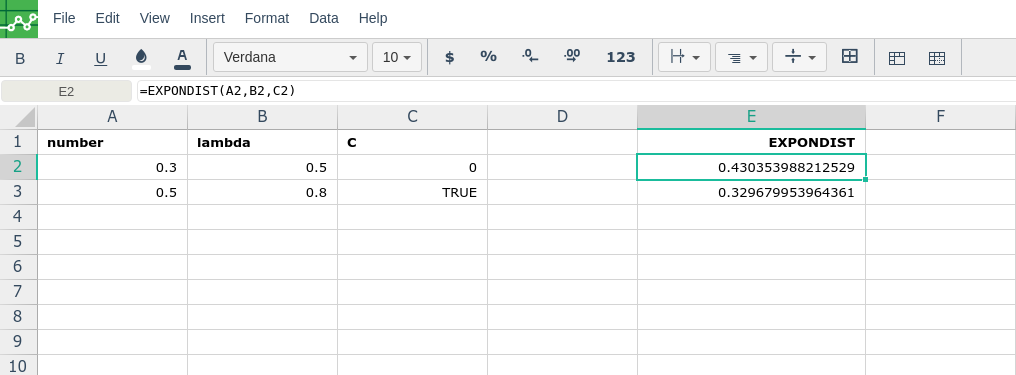# EXPONDIST

### Description

Returns the value of the exponential distribution function with a specified lambda at a specified value.

### Syntax

`EXPONDIST(x, lambda, [cumulative])`

• `x` - The input to the exponential distribution function.

• If `cumulative` is `TRUE` then `EXPONDIST` returns the cumulative probability of all values up to `x`.
• `lambda` - The lambda to specify the exponential distribution function.

• `cumulative` - [ OPTIONAL - `FALSE` by default ] - Whether to use the exponential cumulative distribution.

`WEIBULL`: Returns the value of the Weibull distribution function (or Weibull cumulative distribution function) for a specified shape and scale.

`POISSON`: Returns the value of the Poisson distribution function (or Poisson cumulative distribution function) for a specified value and mean.

`NORMSINV`: Returns the value of the inverse standard normal distribution function for a specified value.

`NORMSDIST`: Returns the value of the standard normal cumulative distribution function for a specified value.

`NORMINV`: Returns the value of the inverse normal distribution function for a specified value, mean, and standard deviation.

`NORMDIST`: Returns the value of the normal distribution function (or normal cumulative distribution function) for a specified value, mean, and standard deviation.

`NEGBINOMDIST`: Calculates the probability of drawing a certain number of failures before a certain number of successes given a probability of success in independent trials.

`LOGNORMDIST`: Returns the value of the log-normal cumulative distribution with given mean and standard deviation at a specified value.

`LOGINV`: Returns the value of the inverse log-normal cumulative distribution with given mean and standard deviation at a specified value.

`HYPGEOMDIST`: Calculates the probability of drawing a certain number of successes in a certain number of tries given a population of a certain size containing a certain number of successes, without replacement of draws.

`BINOMDIST`: Calculates the probability of drawing a certain number of successes (or a maximum number of successes) in a certain number of tries given a population of a certain size containing a certain number of successes,

with replacement of draws.

###Error.

User does not have sufficient privileges to access this Content